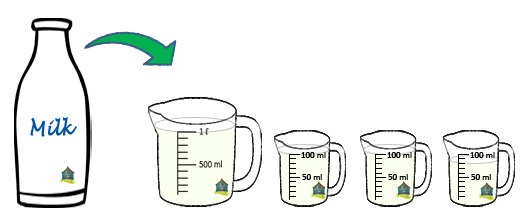## Litres and Millilitres

Practice Unlimited Questions

#### What are litre (liter) and millilitre (milliliter)?

Litre (l) and millilitre (ml) are units of volume and capacity.

1 l  =  1000 ml
Or,
1000 ml  =  1 l

#### 1. Angela has a bottle filled with milk to the brim. She empties the bottle into the following measuring cups. Find the capacity of the bottle in litres and millilitres.Volume of milk in the 1st cup = 1000 ml
Volume of milk in the 2nd cup = 100 ml
Volume of milk in the 3rd cup = 100 ml
Volume of milk in the 4th cup = 80 ml

Total volume of milk in the 4 cups
=  1000 ml + 100 ml + 100 ml + 80 ml
=  1280 ml
=  1 l 280 ml

The capacity of the bottle is 1 l 280 ml.

#### 2. A container has 1 l 625 ml of juice. Express the volume of juice in the container in millilitres.

The amount of juice in a container is called the volume of juice in the container.
We know,
1 l  =  1000 ml

1 l 625 ml
=  1 l  +  625 ml
=  1000 ml  +  625 ml
=  1625 ml

The volume of juice in the container is 1625 ml.

#### 3. A flask has 4 l 50 ml of chemical in it. Express the volume of chemical in the flask in millilitres.

We know,
1 l  =  1000 ml

So,
4 l  =  4000 ml

Hence,
4 l 50 ml
=  4 l  +  50 ml
=  4000 ml  +  50 ml
=  4050 ml

The volume of chemical in the flask is 4050 ml.

#### 4. Mr. Thomas filled 3004 ml of water in a pail. Find the volume of water in the pail in litres and millilitres.

We know,
1000 ml  =  1 l

So,
3000 ml  =  3 l

Hence,
3004 ml
=  3000 ml  +  4 ml
=  3 l  +  4 ml
=  3 l 4 ml

Volume of water in the pail is 3 l 4 ml.